## Power (Triangle)

The total Power of a Triangle is defined by(1)

where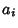are the side lengths, and the partial power'' is defined by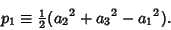(2)

Then(3)(4)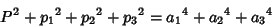(5)(6)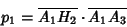(7)(8)(9)

whereis the Area of the Triangle and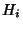are the Feet of the Altitudes. Finally, if a side of the Triangle and the value of any partial power are given, then the Locus of the third Vertex is a Circle or straight line.